Courses

# Hydrology

## 25 Questions MCQ Test GATE Civil Engineering (CE) 2022 Mock Test Series | Hydrology

Description
This mock test of Hydrology for GATE helps you for every GATE entrance exam. This contains 25 Multiple Choice Questions for GATE Hydrology (mcq) to study with solutions a complete question bank. The solved questions answers in this Hydrology quiz give you a good mix of easy questions and tough questions. GATE students definitely take this Hydrology exercise for a better result in the exam. You can find other Hydrology extra questions, long questions & short questions for GATE on EduRev as well by searching above.
QUESTION: 1

### The return period for the annual maximum flood of a given magnitude is 8 years. The probability that this flood magnitude will be exceeded once during the next 5 years is :

Solution: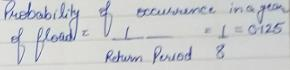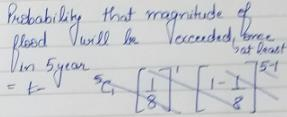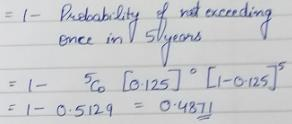QUESTION: 2

Solution:
QUESTION: 3

### The flood hydrograph due to rainfall of intensity 1 cm/h is given by Q = 2 - (1 + t) e-2t (t is in hours and Q is in m3/s). Find  the area of catchment ?

Solution: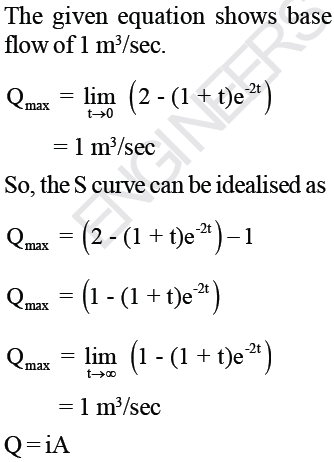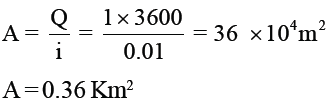*Answer can only contain numeric values
QUESTION: 4

The average surface area of the reservoir in the month of April is 25 Km2. The average rate of inflow is 10 m3/s and outflow rate is 16 m3/s, monthly rainfall is 10 cm, monthly seepage loss is 1.8 cm and he change in storage is 20 million m3. The evaporation (in cm) in that month is :___________

(Important - Enter only the numerical value in the answer)

Solution: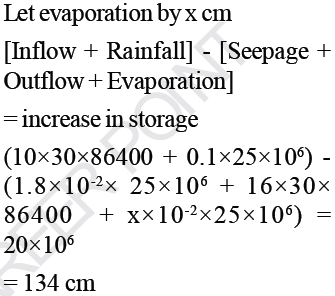*Answer can only contain numeric values
QUESTION: 5

The number of revolution of a current meter is 50 seconds were found to be 12 and 30 corresponding to the velocities of 0.25 m/s and 0.46 m/s respectively. What velocity (in m/s) would be indicated by 50 revolutions of that current meter in 1 minute ?

(Important - Enter only the numerical value in the answer)

Solution: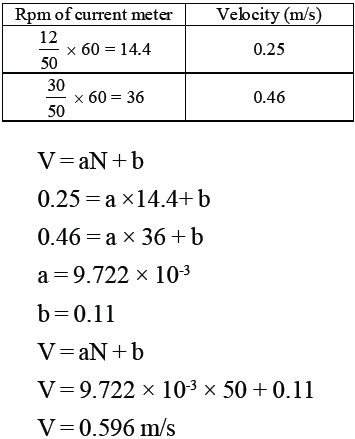QUESTION: 6

The probability that a 10 year return period flood will occur atleast once in the next 4 year is :

Solution: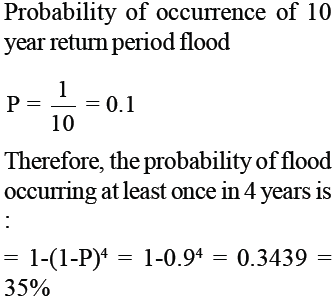QUESTION: 7

If the base period of a 6 hr unit hydrograph (UH) of a basin is 84 hours, then a 12 hr unit hydrograph derived from this 6 hr unit hydrograph will have a base period of :

Solution:

To obtain 12 hr UH, we lag 6hr UH by another 6 hours hence, base period of 12 hr UH is 84 + 6 = 90 hr.

QUESTION: 8

A 6 hr storm has 6 cm of rainfall and the resulting runoff of 3 cm. If φ index remains at the same value,
which one of the following is the runoff due to 12 cm of rainfall in 9 hr in the catchment ?

Solution: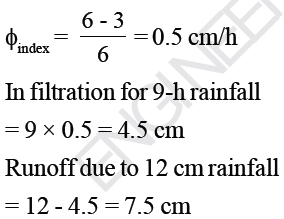QUESTION: 9

Linked Questions 09 and 10

The drainage area of a watershed is 50 Km2. The φ-index is 0.5 cm/hr and the base flow at the outlet is 10m3/s. One hour unit hydrograph of a watershed is triangular in slope with the time base of 20 hr. The
peak ordinate occurs at 10 hours. 9.

Q. The peak ordinate (in m3/s) of the unit hydrograph is :

Solution: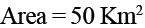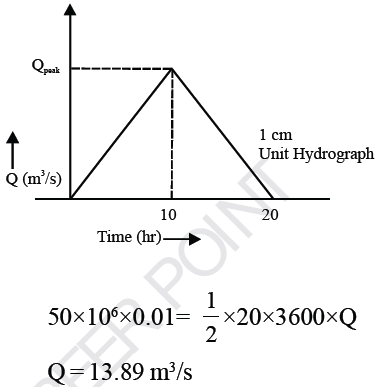QUESTION: 10

Linked Questions 09 and 10
The drainage area of a watershed is 50 Km2. The φ-index is 0.5 cm/hr and the base flow at the outlet is 10m3/s. One hour unit hydrograph of a watershed is triangular in slope with the time base of 20 hr. The peak ordinate occurs at 10 hours.

Q. For the storm of depth 6.5 cm and duration 1 hr, the peak ordinate (in m3/s) of the flood hydrograph is :

Solution: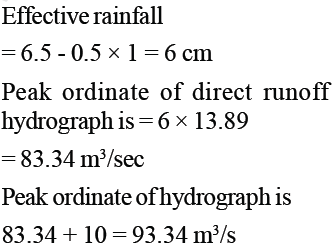QUESTION: 11

A 3 hour storm over a water shed had an average depth of 27mm. The resulting flood hydrograph was found to have a peak flow of 200 m3/s and a base flow of 20 m3/s. If the loss rate could be estimated as 0.3 cm/h, a 3-h unit hydrograph for this water shed will

Solution: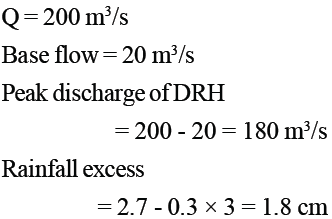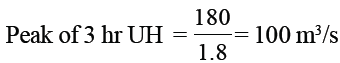QUESTION: 12

The 3 hr unit hydrograph U1 of a catchment of area 250 km2 is in the form of a triangle with peak discharge of 40 m3/s. Another 3 hour unit hydrograph U2 is also  triangular in shape and has the same base width as U1 but with a peak flow of 80m3/s. The catchment which U2 refers to has an area of :

Solution: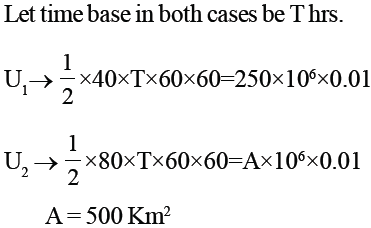QUESTION: 13

The storm hydrograph was due to 3h of effective rainfall. It contained 6cm of direct runoff. The ordinates of DRH of this storm :

Solution:
QUESTION: 14

The basic assumptions of the unit hydrograph theory are :

Solution:
QUESTION: 15

Depth-Area-Duration curves of precipitations are drawn as :

Solution:
QUESTION: 16

The return period that a designs must use in the estimation of a flood for a hydraulic structure, if he is willing to accept 20% risk that the flood of that or higher magnitude will occur in the next 10 year, is

Solution: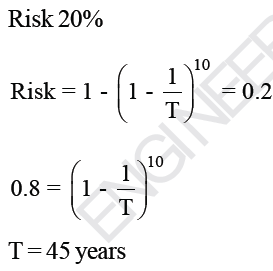QUESTION: 17

The standard project flood will generally be :

Solution:
QUESTION: 18

In a Linear reservoir, the

Solution:
*Answer can only contain numeric values
QUESTION: 19

A reservoir with surface area of 250 hectares has saturation vapour pressure at water surface = 17.54 mm of Hg and actual vapour pressure of air = 7.02mm of Hg. Wind velocity at 1m above the ground surface = 16 km/hr. Estimate the average daily evaporation from the lake using Meysis formula……….mm/days.
(Take KM = 0.36)

Solution: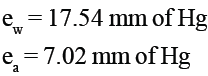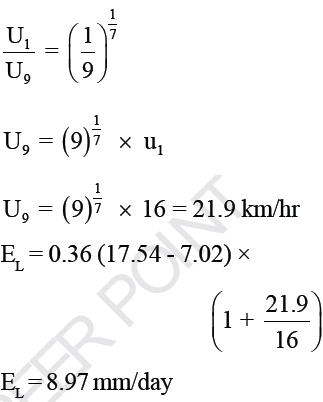QUESTION: 20

How is the average velocity along the vertical in a wide stream obtained?

Solution:

Average velocity in a wide stream can be calculated by measuring the velocity at half the depth.
Average velocity in a deep stream can be calculated by averaging the velocity at 0.2 and 0.8 times depth from surface.

QUESTION: 21

The intensity of rainfall time interval of a typical storm are :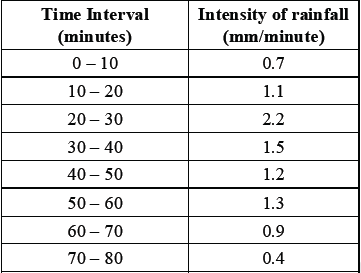The maximum intensity of rainfall for 20 minute duration of storm is:

Solution:
QUESTION: 22

The coefficient of variation of the rainfall for six rain gauge stations in a catchment was found to be 29.54% The optimum number of stations in the catchment for an admissible 10% error in the estimation of mean rainfall will be:

Solution: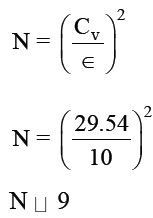QUESTION: 23

A 4hr storm had 4 cm of rainfall and the resulting direct runoff was 2 cm. If the φ-index remain at the same value, the runoff due to 10 cm of rainfall in 8 hr in the catchment is ?

Solution: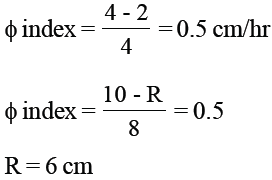QUESTION: 24

Consider the following chemical emulsions :

1. Methyl Alcohol

2. Cetyl Alcohol

3. Stearye Alcohol

4. Kerosene

Which of the above chemical emulsions is/are used to minimize the loss of water through the process of evaporation ?

Solution:
QUESTION: 25

Which of the following defines Aridity Index (AI) ?

Solution: